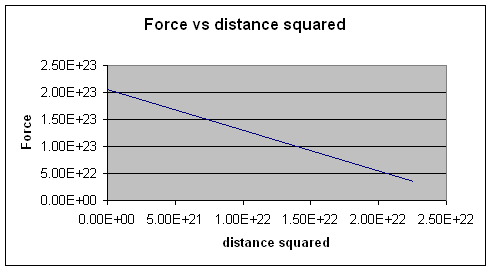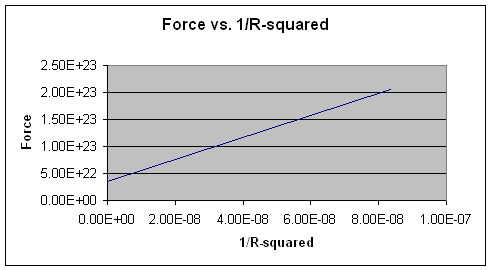#### Lab: Confirm that Newton's Law of Universal Gravitation  is an inverse square lawGiven: the following data

 Average Earth -Moon Distance (m) Radius of Moon(m) Radius of Earth(m) Mass of Earth(kg) Mass of Moon(kg) Mass of Sun(kg) Average Earth -Sun Distance (m) Radius of the Sun(m) 3.84 x 10 6 1.74 x 106 6.38 x 106 5.98 x 1024 7.35 x 1022 1.99 X 10 30 1.496 x 10 11 6.96 x 108

Task: use graphical techniques to confirm that  Newton's Law of Universal Gravitation  is an inverse square law.

Solution:Note: The center-to-center distance between the Moon and the Earth is the distance between the two celestial bodies plus their respective radii.  i.e.

For The Moon -Earth System

R1 = 1.74 x 10m + 6.38 x 106  m + 3.84 x 10 m  = 1.196 x 10 m

For the Sun -Earth System

R= 1.496 x 1011 m + 6.38 x 106  m + 6.96 x 10 m  = 1.50 x 10 11 m

Procedure:

Use the Equation for the Law of Universal Gravitation1. Find the forces for Moon -Earth System
F= 2.05 x 1023 N
2. Find the forces for the Sun -Earth System
F2 = 3.53 x 1022 N
3. Compile the data in a chart and Plot the data on a graph
 System Forces (N) Distances (R)(m) Distances-squared (R)2 , (m2) Product of Masses(kg2) Earth-Moon 2.05 x 1037 1.196 x 10 7 1.430 x 10 14 4.57 x 1047 Earth-Sun 3.53 x 1022 1.50 x 10 11 2.25  x 10 22 1.19 x 1055Here is the same data and its analysis using spreadsheets

 System Forces (N) Distances (R) Distances-squared (R)2 , (m2) Product of Masses Inverse Distances-squared (1/R)2 , (1/m2) (m) (kg2) Earth-Moon 2.05E+37 1.20E+07 1.43E+14 4.57E+47 6.99E-15 Earth-Sun 3.53E+22 1.50E+11 2.25E+22 1.19E+55 4.44E-23This shows that in the relationship F α 1/R2,Force is inversely proportional to the distance squaredTo confirm the inverse square relationship we can plot F vs. 1/R2Note: F vs. 1/R2 gives us  a linear relationship.

Conclusion: the Law of Universal Gravitation is a an inverse square law